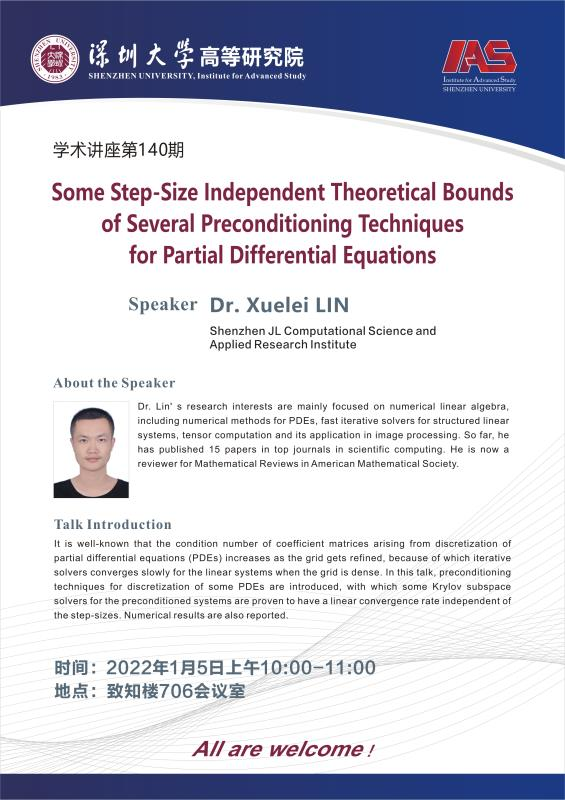﻿ 高等研究院系列学术讲座一百四十-深圳大学高等研究院

高等研究院系列学术讲座一百四十

Some Step-Size Independent Theoretical Bounds of Several Preconditioning Techniques for Partial Differential Equations

Speaker Dr. Xuelei LIN

Shenzhen JL Computational Science and Applied Research Institute

Dr. Lin’s research interests are mainly focused on numerical linear algebra, including numerical methods for PDEs, fast iterative solvers for structured linear systems, tensor computation and its application in image processing. So far, he has published 15 papers in top journals in scientific computing. He is now a reviewer for Mathematical Reviews in American Mathematical Society.

Talk Introduction

It is well-known that the condition number of coefficient matrices arising from discretization of partial differential equations (PDEs) increases as the grid gets refined, because of which iterative solvers converges slowly for the linear systems when the grid is dense. In this talk, preconditioning techniques for discretization of some PDEs are introduced, with which some Krylov subspace solvers for the preconditioned systems are proven to have a linear convergence rate independent of the step-sizes. Numerical results are also reported.

All are welcome！